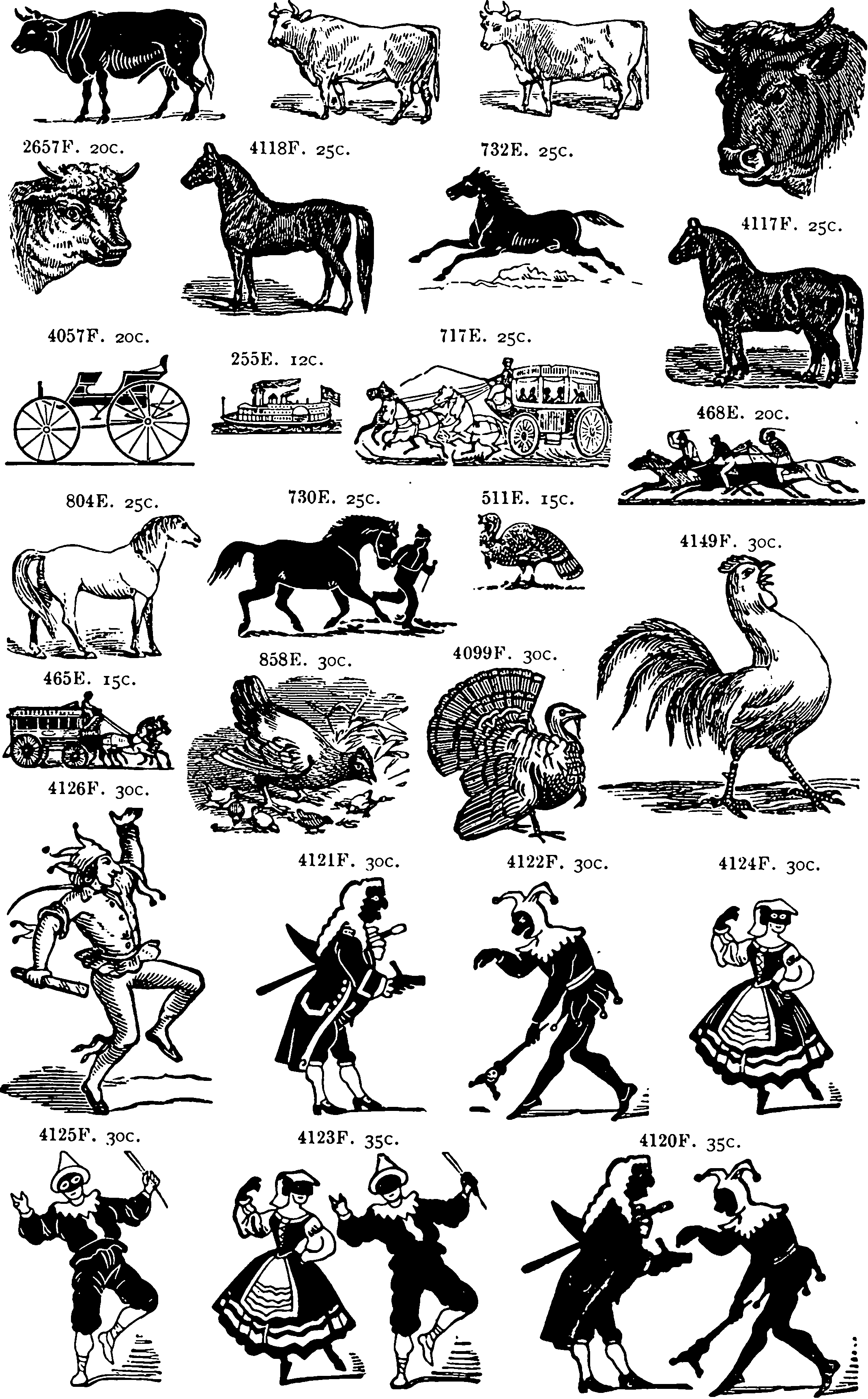# Pólya-Gamma augmentation trickAn infinite weird RV useful Bayesian Binomial regression (and maybe other things?) . C&C the optimization-driven approach to a similar problem in Gumbel-max tricks.

See also my former colleague, Louis Tiao, A Primer on Pólya-gamma Random Variables - Part II: Bayesian Logistic Regression.

Gregory Gunderson, in Pólya-Gamma Augmentation, explains the problem we are trying to solve.

…n logistic regression, the dependent variables are assumed to be i.i.d. from a Bernoulli distribution with parameter $$p$$, and therefore the likelihood function is $\mathcal{L}(p) \propto \prod_{n=1}^{N} p^{y_{n}}(1-p)^{1-y_{n}}=p^{\sum y_{n}}(1-p)^{N-\sum y_{n}}$ The observations interact with the response through a linear relationship with the log-odds, $\log \left(\frac{p}{1-p}\right)=\beta_{0}+x_{1} \beta_{1}+x_{2} \beta_{2}+\cdots+x_{D} \beta_{D}=\beta^{\top} \mathbf{x}$ If we solve for $$p$$ in (2), we get $p=\frac{\exp \left(\boldsymbol{\beta}^{\top} \mathbf{x}_{n}\right)}{1+\exp \left(\boldsymbol{\beta}^{\top} \mathbf{x}_{n}\right)}$ and a likelihood of $\mathcal{L}(\boldsymbol{\beta}) \propto \frac{\left[\exp \left(\boldsymbol{\beta}^{\top} \mathbf{x}\right)\right]^{\sum y_{n}}}{\left[1+\exp \left(\boldsymbol{\beta}^{\top} \mathbf{x}\right)\right]^{N}}$ Due to this functional form, Bayesian inference for logistic regression is intractable.

Using Pólya-Gamma RVs we devise an auxiliary variable sample.

### No comments yet. Why not leave one?

GitHub-flavored Markdown & a sane subset of HTML is supported.